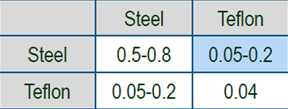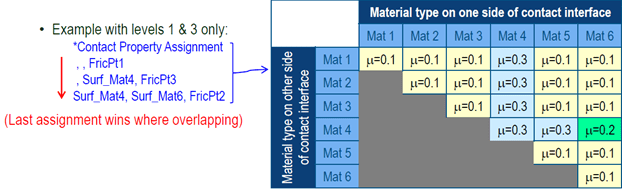## Combinatorial method in Abaqus friction modeling

In this article we are going to discuss an advanced friction modeling technique in Abaqus. It is based on combination rules that allows solver to compute effective friction interaction based on two contacting surfaces with different coefficients of friction. As an example, look at the following table:If someone asks: “what is the coefficient of friction of steel?” There really is no answer to this question. The answer really depends on the other object with which steel interacts. The table shows two different values, one for steel-steel interaction and other with steel-teflon interaction. If the user has NXM matrix of materials interacting with each other and each cell of that matrix has a friction coefficient assigned to it, then modeling in Abaqus is trivial. Define surface interaction with friction coefficient for each cell and use it with corresponding surface pair in the contact property assignment. The example below highlights it.But this straightforward approach is possible only if friction values for all cells are available. However, at times only the diagonal values are available. That means all the non-diagonal cell values are unknown. In that case contact property assignment is not possible.

Abaqus now allows users to define friction as surface property as well. For two different surfaces (A,B) with individual coefficient of friction, the effective friction for pair is computed as follows:The default value of alpha is 0.3. In case of mixed problems, where surface property and contact property methods co-exist, either method can take precedence. Look at following example.The approach is an approximation but its worth in situations where user has no access to friction coefficients values for all the contact material pairs. This friction algorithm is available in Abaqus explicit 2018 release and beyond.